# Line Segment - Definition with Examples

The Complete K-5 Math Learning Program Built for Your Child

• 30 Million Kids

Loved by kids and parent worldwide

• 50,000 Schools

Trusted by teachers across schools

• Comprehensive Curriculum

Aligned to Common Core

## What is Line Segment?

This is a line! It has no endpoints and extends endlessly in both directions.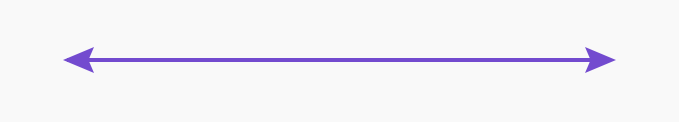If you mark two points A and B on it and pick this segment separately, it becomes a line segment.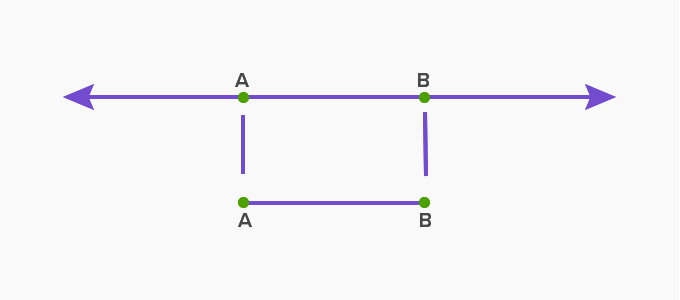This line segment has two endpoints A and B whose length is fixed. The length of this line segment is the distance between its endpoints A and B.

So, a line segment is a piece or part of a line having two endpoints. Unlike a line, a line segment has a definite length.

The length of a line segment can be measured either in metric units such as millimeters, centimeters, or customary units like feet or inches.

### Measuring a line segment

Follow the given steps to measure the length of a line segment:

Step 1: Pick up a scale to measure the length of a line segment. Generally smaller line segments are measured using a centimeter scale.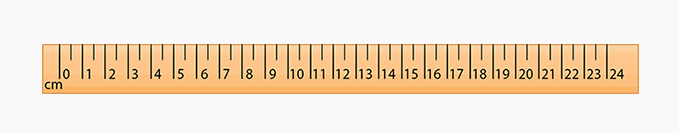Step 2: Identify the line segment you want to measure.Step 3: Place the tip of the ruler at the starting point of the line segment.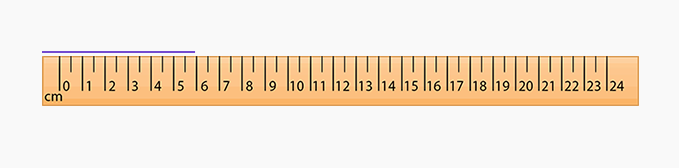Step 4: Read the number on the scale where the line segment ends. In this case, it is 5. So, the length of the given line segment is 5 cm.

### Drawing a line segment using ruler and compass

Suppose we need to draw a line segment of length 5 cm. We will follow the given steps:

• Step 1: Draw a line of any length. Mark a point A on the line, which is the starting point of the line segment.

• Step 2: Using a ruler, place the pointer of the compass 5 cm apart from the pencil’s lead.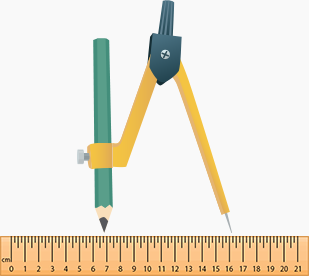• Step 3: Place the pointer of the compass at A and mark an arc on the line with the pencil point.

• Step 4: Mark the point where the arc and the line intersect as B.

• Step 5: AB is the required line segment of length 5 cm.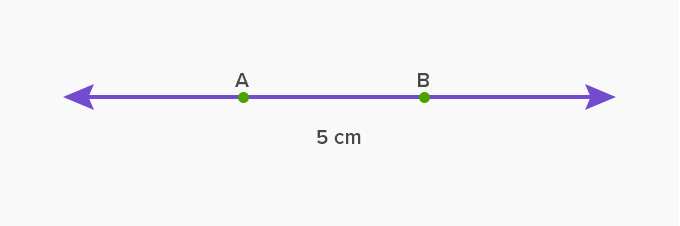### Real life example

Sides of a polygon, edges of a ruler, edges of a paper are all examples of a line segment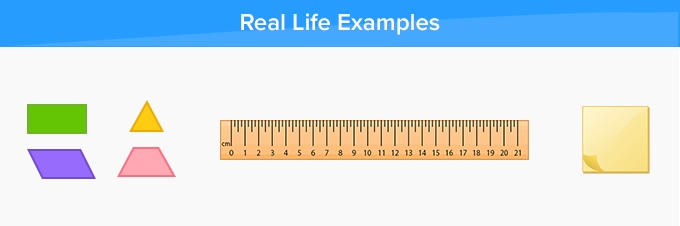Fun Facts about line segment A line segment is made up of two words line and segment. The line has the origin of Latin, Old English, and Old French which means rope or series and segment comes from Latin Segmentum which means strip, a piece cut off, or segment of earth.

Won Numerous Awards & Honors